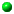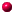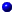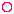Arithmetic is the study of numbers.

Arithmetic and practical geometry are the oldest parts of mathematics

The four basic parts of arithmetic are:
Subtraction
Multiplication
Division

These are called the four basic operations of arithmetic. They are also the four basic operations of algebra.

Addition: To calculate the total of two or more numbers.
For example: 2 + 3 = 5.
The total is called the sum of the numbers added.

Subtraction: To find the difference between two numbers by taking one away from the other. For example:
Subtracting 3 from 7 leaves 4.
Or: 7 - 3 = 4

Multiplication:

Multiplication is a short way to add several of the same numbers. It can be done mentally or on an electronic calculator.

To multiply mentally, you need to know multiplication tables

For example:

To add 3 + 3 + 3 + 3 + 3 + 3 + 3 (which equals 21)
You remember a multiplication table that tells you that:
7 threes are 21.
Or, in symbols, 7 x 3 = 21.

Division: Finding out how many time one number contains another. For example:

15 contains 5 three times.
The 15 is the dividend (The number divided)
The 5 is the divisor (The number dividing)
The result (3) is the quotient

Approximation:

Aproximation is simplifying an answer by rounding it off, when exact accuracy is not required, but quick comprehension is.

For example:

2517 is approximately 2520 or 2500.

Decimals

A decimal is something based on the number ten. It particularly refers to showing parts (fractions) of numbers as tenths by writing numbers on both sides of a point, called a decimal point

For example:

5.5 means five and five tenths (five and a half)
5.9 means five and nine tenths
5.09 means five and nine hundredths
5.59 means five, five tenths and nine hundredthsSee the Decimal System

Digits

In arithmetic, digits are the individual numbers for counting in tens:
0, 1, 2, 3, 4, 5, 6, 7, 8 and 9.

Your digits are your fingers, thumbs and toes. If we take our socks off, we can count to twenty on them. It is more convenient, however, to just use the digits on our hands. This gives us ten as a basic unit for counting. By turning down a digit every time we get to ten, we can count to one hundred.

Fraction

The word fraction means a broken bit. If you fractured a saucer in two you would have two fractions of the saucer. If the two pieces were the same size, the fractions would be halves.

The top number in a fraction is called the numerator

The bottom number in a fraction is called the denominator

A fraction is part of a whole

A factor, or divisor, of a (whole) number is one which divides exactly into it. For example, 3 is a factor of 12 because four 3s are 12.

All numbers have 1 as a factor. Numbers apart from one have other factors as well.

Example:

A multiple is a number that can be divided exactly by another. For example, 12 is a multiple of 3 because 12 can be divided by 3 four times, leaving no remainder.

The lowest common multiple or least common multiple is the smallest number that is a multiple of a group of numbers. This is the same as saying, the smallest number into which every one of a group of numbers will divide exactly.

For example:

30 is the lowest common multiple of 3, 5 and 6.

Lowest Common Denominator or Least Common Denominator
The
lowest common multiple of the denominators of several fractions

For example:

If the fractions are: ¼ ½ and ¾

Their denominators (bottom line) are 4, 2 and 4

4 is the lowest common denominator

Lowest Common Denominator is sometimes used as a metaphor. When a report on study skills spoke of students working towards the "lowest common denominator", it appears to have meant that if students can get away with something in one module, they will try to get away with it in another.

Interest
Money that you pay in exchange for borrowing money.
Or (the other way round) money that someone pays you in exchange for borrowing your money.

The money that someone lends is called the capital.
The interest is usually expressed as a
percentage of the capital.
The interest added to the capital makes a sum of money called the amount.

In simple interest the capital remains the same each year and so the interest also remains the same.

In compound interest the interest at the end of each year is added to the capital, and so the interest increases from year to year.

Percentage
 Per cent means [parts] per hundred. So five per cent means five in every hundred Five per cent can be written as 5% 5% five in every hundred

If A and B are any two numbers, A is A/B x 100 percent of B

If a number is part of a total and you want to calculate what percentage it is, you divide the number by the total and multiply by 100.

 number ----- total This can be expressed as the formula: (n/t) x 100

For example, if there are 100 students taking an exam and 17 chose a question from the organic chemistry section, it is clear that 17% chose an organic chemistry question.

17/100 = .17
.17 x 100 = 17%

If 113 students take an exam and 17 chose a question from the organic chemistry section, it is not self evident what the percentage choosing an organic chemistry question is. But the formula calculates it:

17/113 = .15
.15 x 100 = 15%

Percentage change or percentage difference
may be a percentage increase or a percentage decrease

The change (increase or decrease) in a value is expressed as a percentage of the original value.

If the price of something increases from 100 pence to 110 pence, there is a 10% increase.

To calculate the change you need the difference between the original and final figure.

110 - 100 = 10
10/100 = .1
.1 x 100 = 10

Or (when it is not so obvious)

£79.86 is increased to £91.22 the increase is what percent?

91.22 - 79.86 = 11.36
11.36/79.86 = 14.22 [I used a calculator for that]

So the percentage increase is 14.22%

Thank you Nicola Thompson for asking the question

Progression

Progression, like progress, is about moving from where you are by steps.

In mathematics, a progression is a series of numbers that increases or decreases by steps. The steps are proportional to one another. Different kinds of progression have different proportions.

Arithmetic Progression
An example of an arithmetic progression is:

1, 4, 7, 10, 13, 16, 19, 22, 25.....

Geometric Progression
An example of geometric progression is:

1, 4, 16, 64, 256, 1,024.....

Proportion
The proportion or ratio that one number bears to another is the result of dividing one by the other.

For example,
The proportion of 3 to 7 is three divided by seven = 0.42
The proportion of 6 to 14 is six divided by fourteen
This is also 0.42

Any number divided into itself is one. So the ratio of a quantity to the same quantity of something else is one. Look at the graph of the British balance of trade. This has the ratio of export to import volumes as its vertical axis. When exports and imports are equal, the ratio is one.

Wikipedia: rate -

A rate is often a ratio: the quantity of something expressed as a proportion. For example, Durkheim defines the rates of births, marriages, and suicides as

" the number obtained by dividing the average annual total of marriages, births, suicides, by the number of persons whose ages lie within the range in which marriages, births, and suicides occur."

Parsons distinguishes between rates and incidence

A frequent use of rates in statistics is to construct time series showing the rate of change over timeStudy links outside this siteAndrew Roberts' web Study GuidePicture introduction to this siteTop of PageTake a Break - Read a PoemClick coloured words to go where you want

Andrew Roberts likes to hear from users:
To contact him, please use the Communication Form

Maths index

*****************

Arithmetic index

